# 机器学习公式推导

本篇笔记主要记录及推导Andrew NG的Machine Learning课程中出现的公式。

我们假设对于任意的分类、聚类、回归等问题在自然界中总是存在一个精确的模型与之相对应，接下来我们要做的就是根据获取的样本来反推并确定这个模型。由于我们毕竟无法遍历这个问题所有的情况，所以我们只能根据获取的样本去尽可能接近的确定这个模型。

公式化上面这段描述，问题对应的模型就藏在假设空间(Hypothesis) ${h}_{\theta }\left(x\right)$$h_\theta(x)$中，我们需要通过观测样本，确定其中的$\theta$$\theta$值。在确定$\theta$$\theta$值的过程中，定义一个损失函数(Cost Function) $J\left(\theta \right)$$J(\theta)$，如果我们获取的样本在某一个参数$\theta$$\theta$时使损失值达到最小，即表示当前$\theta$$\theta$值确定的模型可以使预测值很接近观察值。那么这个模型就是我们需要寻找的。

对于监督学习，我们要做的就是确定目标函数，损失函数，然后通过样本训练，得到损失值最小的那一组参数值，用该参数值代入目标函数，即可得到对应问题的模型。

# 一、线性回归模型

## 1、单一变量的线性回归模型

目标函数

${h}_{\theta }\left(x\right)={\theta }_{0}+{\theta }_{1}x$

损失函数
$J\left({\theta }_{0},{\theta }_{1}\right)=\frac{1}{2m}\sum _{i=1}^{m}\left({h}_{\theta }\left({x}^{\left(i\right)}\right)-{y}^{\left(i\right)}{\right)}^{2}$

公式说明：
${h}_{\theta }\left({x}^{\left(i\right)}\right):第i个样本$$h_\theta(x^{(i)}):第i个样本$
${y}^{\left(i\right)}:第i个样本对应的实际值$$y^{(i)}:第i个样本对应的实际值$

接下来的目标就是找到一组参数值，使得损失函数值最小，即

$\underset{{\theta }_{0},{\theta }_{1}}{minimize}J\left({\theta }_{0},{\theta }_{1}\right)$

求损失函数最小值时，使用梯度下降(Gradient descent)的方法。在微积分中我们学过梯度，梯度方向是函数值下降最快的方向，所以在梯度下降方法中，我们分别求${\theta }_{0}和{\theta }_{1}$$\theta_0和\theta_1$的偏导数，然后用该导数值更新参数值。

说明，上面公式中的$:=$$:=$表示赋值的意思，如果直接写a = 1可能会被误理解为判断a是否等于1

求损失函数$J\left({\theta }_{0},{\theta }_{1}\right)$$J(\theta_0, \theta_1)$${\theta }_{0}$$\theta_0$${\theta }_{1}$$\theta_1$的偏导数，

$\frac{\mathrm{\partial }}{\mathrm{\partial }{\theta }_{0}}J\left({\theta }_{0},{\theta }_{1}\right)=\frac{1}{m}\sum _{i=1}^{m}\left({h}_{\theta }\left({x}^{\left(i\right)}\right)-{y}^{\left(i\right)}\right)\phantom{\rule{0ex}{0ex}}\frac{\mathrm{\partial }}{\mathrm{\partial }{\theta }_{1}}J\left({\theta }_{0},{\theta }_{1}\right)=\frac{1}{m}\sum _{i=1}^{m}\left({h}_{\theta }\left({x}^{\left(i\right)}\right)-{y}^{\left(i\right)}\right){x}^{\left(i\right)}$

## 2、多变量线性回归模型

上一节的模型中只有一个指标$x$$x$，理解了线性回归模型及其寻找最优化参数的过程。接下来将该思路应用到多变量模型中。

### （1）目标函数

${h}_{\theta }\left(x\right)={\theta }_{0}{x}_{0}+{\theta }_{1}{x}_{1}+{\theta }_{2}{x}_{2}+\cdots +{\theta }_{n}{x}_{n}$

上式中的${x}_{1},{x}_{2},\cdots ,{x}_{n}$$x_1, x_2, \cdots, x_n$都是给定样本中的指标，其中${x}_{0}=1$$x_0 = 1$是人为增加的。
如果将目标函数使用向量表示，
${h}_{\theta }\left(x\right)={\theta }^{T}x$

### （2）损失函数

$J\left(\theta \right)=\frac{1}{2m}\sum _{i=1}^{m}\left({h}_{\theta }\left({x}^{\left(i\right)}\right)-{y}^{\left(i\right)}{\right)}^{2}$

### （3）梯度下降

分别对${\theta }_{0},{\theta }_{1},{\theta }_{2}$$\theta_0, \theta_1, \theta_2$求偏导数并进行展开，如下所示

${\theta }_{0}:={\theta }_{0}-\alpha \frac{1}{m}\sum _{i=1}^{m}\left({h}_{\theta }\left({x}^{\left(i\right)}\right)-{y}^{\left(i\right)}\right)\phantom{\rule{0ex}{0ex}}{\theta }_{1}:={\theta }_{1}-\alpha \frac{1}{m}\sum _{i=1}^{m}\left({h}_{\theta }\left({x}^{\left(i\right)}\right)-{y}^{\left(i\right)}\right){x}_{1}^{\left(i\right)}\phantom{\rule{0ex}{0ex}}{\theta }_{2}:={\theta }_{2}-\alpha \frac{1}{m}\sum _{i=1}^{m}\left({h}_{\theta }\left({x}^{\left(i\right)}\right)-{y}^{\left(i\right)}\right){x}_{2}^{\left(i\right)}\phantom{\rule{0ex}{0ex}}\cdots$

### （4）公式法

如果我们将目标函数向量化，$T{X}^{T}\theta =y$$TX^T\theta=y$，需要求解其中的$\theta$$\theta$

$X\theta =y\phantom{\rule{0ex}{0ex}}{X}^{T}X\theta ={X}^{T}y\phantom{\rule{0ex}{0ex}}\theta =\left({X}^{T}X{\right)}^{-1}{X}^{T}y$

这里需要说明一下，$\theta ,y,X$$\theta, y, X$分别代表的含义。在本文中，向量都是小写字母表示，并且都是列向量，即$n\ast 1$$n *1$维。矩阵的维度$m\ast n$$m * n$表示有$m$$m$$n$$n$列。那么上式中，我们假设$m=4$$m=4$$n=5$$n=5$其中包括${x}_{0}$$x_0$，给出一组示例数据

$\begin{array}{clcrrr}{x}_{0}& {x}_{1}& {x}_{2}& {x}_{3}& {x}_{4}& y\\ 1& 2104& 5& 1& 45& 460\\ 1& 1416& 3& 2& 40& 232\\ 1& 1534& 3& 2& 30& 315\\ 1& 852& 2& 1& 36& 178\end{array}$

对应的$X$$X$为，每个${x}^{\left(i\right)}$$x^{(i)}$表示一行数据的话：

$X=\left[\begin{array}{ccccc}1& 2104& 5& 1& 45\\ 1& 1416& 3& 2& 40\\ 1& 1534& 3& 2& 30\\ 1& 852& 2& 1& 36\end{array}\right]=\left[\begin{array}{c}\left({x}^{\left(1\right)}{\right)}^{T}\\ \left({x}^{\left(2\right)}{\right)}^{T}\\ \left({x}^{\left(3\right)}{\right)}^{T}\\ \left({x}^{\left(4\right)}{\right)}^{T}\end{array}\right]$

对应的$y$$y$为：

$y=\left[\begin{array}{c}460\\ 232\\ 315\\ 178\end{array}\right]$

对应的$\theta$$\theta$为：

$\theta =\left[\begin{array}{c}{\theta }_{0}\\ {\theta }_{1}\\ {\theta }_{2}\\ {\theta }_{3}\end{array}\right]$

# 二、逻辑回归模型

## 1、逻辑回归模型

上面的线性回归模型输出结果为连续值，如果我们面对的是一个分类模型，比如判断是否为垃圾邮件，或者其他的分类问题时，就不能直接使用线性回归模型了。

逻辑回归模型是在线性回归模型上的一个演变，它通过一个逻辑函数可以将线性回归模型的输出结果转变为0或1的离散输出。

### （1）逻辑函数

即Logistic Function，也称为Sigmoid Function，如下所示，

$g\left(z\right)=\frac{1}{1+{e}^{-z}}$

对应的函数图形为：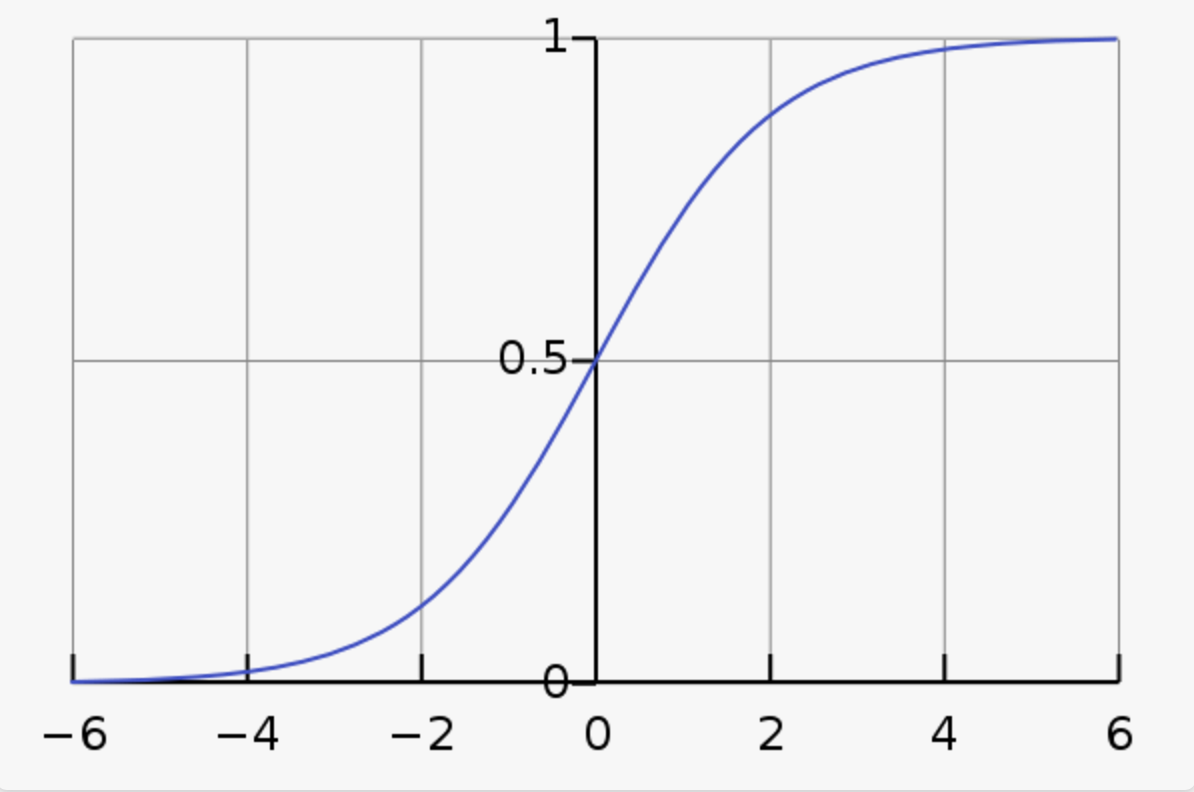从图中可以看到，横轴是连续取值，但是纵轴上的取值范围被限制在0和1之间，Sigmoid函数可以将连续值转变为0或1的离散值。

如果将上面的逻辑函数$g\left(z\right)$$g(z)$应用在线性回归模型的输出函数${h}_{\theta }\left(x\right)$$h_\theta(x)$上，就可以得到本节所讲的逻辑回归模型。

### （2）目标函数

${h}_{\theta }\left(x\right)=\frac{1}{1+{e}^{-{\theta }^{T}x}}$

当$y=1$$y=1$时，${h}_{\theta }\left(x\right)$$h_\theta(x)$的值，可以理解为是对当前样本$x$$x$，在参数$\theta$$\theta$的情况下被预测为1的概率。即

$P\left(y=1|x,\theta \right)={h}_{\theta }\left(x\right)=\frac{1}{1+{e}^{-{\theta }^{T}x}}$

### （3）损失函数

在前面的线性回归模型中，损失函数如下

$J\left(\theta \right)=\frac{1}{2m}\sum _{i=1}^{m}\left({h}_{\theta }\left({x}^{\left(i\right)}\right)-{y}^{\left(i\right)}{\right)}^{2}\phantom{\rule{0ex}{0ex}}=\frac{1}{m}\sum _{i=1}^{m}\frac{1}{2}\left({h}_{\theta }\left({x}^{\left(i\right)}\right)-{y}^{\left(i\right)}{\right)}^{2}$

上式第二行中将$\frac{1}{2}$$\frac 12$向后移动到求和项中，如果将求和项中整体定义为

$Cost\left({h}_{\theta }\left(x\right),y\right)=\frac{1}{2}\left({h}_{\theta }\left({x}^{\left(i\right)}\right)-{y}^{\left(i\right)}{\right)}^{2}$

那么线性回归的损失函数可以写成

$J\left(\theta \right)=\frac{1}{m}\sum _{i=1}^{m}Cost\left({h}_{\theta }\left(x\right),y\right)\phantom{\rule{0ex}{0ex}}Cost\left({h}_{\theta }\left(x\right),y\right)=\frac{1}{2}\left({h}_{\theta }\left({x}^{\left(i\right)}\right)-{y}^{\left(i\right)}{\right)}^{2}$

线性回归使用的是平方损失，如果我们直接将平方损失函数应用到逻辑回归模型中，最终得到的$J\left(\theta \right)$$J(\theta)$可能如下图所示，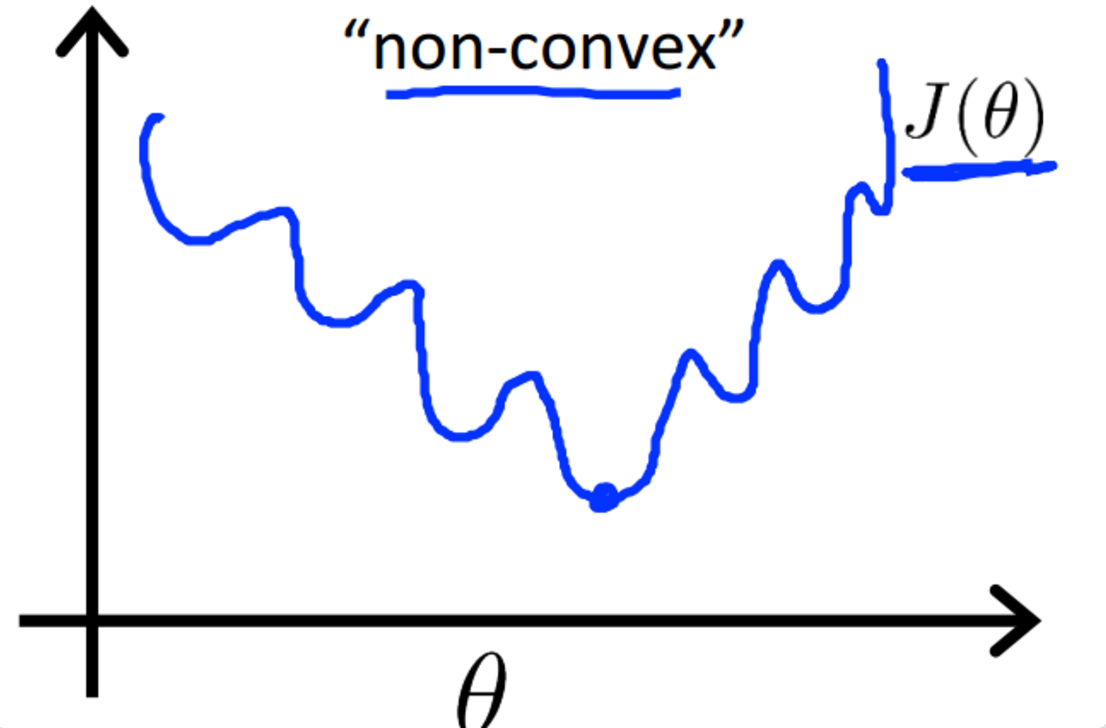逻辑回归模型中使用的是对数损失，定义如下

$Cost\left({h}_{\theta }\left(x\right),y\right)=\left\{\begin{array}{ll}-log\left({h}_{\theta }\left(x\right)\right)& y=1\\ -log\left(1-{h}_{\theta }\left(x\right)\right)& y=0\end{array}$

可以画出对数损失函数图形来看，当$y=1$$y=1$ 并且 ${h}_{\theta }\left(x\right)=1$$h_\theta(x)=1$时，$Cost=0$$Cost=0$，当$y=1$$y=1$ 并且 ${h}_{\theta }\left(x\right)\to 0$$h_\theta(x)\rightarrow0$时，$Cost\to \mathrm{\infty }$$Cost\rightarrow \infty$$y=0$$y=0$时情况类似。

最后，将逻辑回归的对数损失函数进行融合，

$Cost\left({h}_{\theta }\left(x\right),y\right)=-ylog\left({h}_{\theta }\left(x\right)\right)-\left(1-y\right)log\left(1-{h}_{\theta }\left(x\right)\right)$

将$Cost\left({h}_{\theta }\left(x\right),y\right)$$Cost(h_\theta(x), y)$代入$J\left(\mathrm{\Theta }\right)$$J(\Theta)$可以得到逻辑回归完整的损失函数如下

$J\left(\theta \right)=-\frac{1}{m}\sum _{i=1}^{m}\left[{y}^{\left(i\right)}log\left({h}_{\theta }\left({x}^{\left(i\right)}\right)\right)+\left(1-{y}^{\left(i\right)}\right)log\left(1-{h}_{\theta }\left({x}^{\left(i\right)}\right)\right)\right]$

逻辑回归中使用对数损失函数来求解参数，与采用极大似然估计求参数是一致的。

以下为对数损失函数和极大似然估计的分析过程：

假设样本服从伯努利分布(0-1分布)，则有

$P\left({h}_{\theta }\left(x\right)=y\right)=\left\{\begin{array}{ll}1-p& n=0\\ p& n=1\end{array}$

似然函数如下：
$L\left(\theta \right)=\prod _{i=1}^{m}P\left(y=1|{x}_{i},\theta {\right)}^{{y}_{i}}P\left(y=0|{x}_{i},\theta {\right)}^{1-{y}_{i}}$

对数似然函数为：
$lnL\left(\theta \right)=\sum _{i=1}^{m}\left[{y}_{i}ln\left(P\left(y=1|{x}_{i},\theta \right)+\left(1-{y}_{i}\right)lnP\left(y=0|{x}_{i},\theta \right)\right]\phantom{\rule{0ex}{0ex}}=\sum _{i=1}^{m}\left[{y}_{i}ln\left(P\left(y=1|{x}_{i},\theta \right)+\left(1-{y}_{i}\right)ln\left(1-P\left(y=0|{x}_{i},\theta \right)\right)\right]$

根据对数损失函数的定义
$Cost\left(y,p\left(y|x\right)=-ylnp\left(y|x\right)-\left(1-y\right)ln\left(1-p\left(y|x\right)\right)$

那么对于全体样本，损失函数如下：
$Cost\left(y,p\left(y|x\right)=-\sum _{i=1}^{m}\left[{y}_{i}lnp\left({y}_{i}|{x}_{i}\right)-\left(1-{y}_{i}\right)ln\left(1-p\left({y}_{i}|{x}_{i}\right)\right)\right]$

可以看到，对数损失函数与上面的极大似然函数本质上是等价的。所以，逻辑回归直接采用对数损失函数，与采用极大似然估计是一致的。

### （4）梯度下降

接下来使用梯度下降方法求解逻辑回归的最佳参数，求解损失函数

$J\left(\theta \right)=-\frac{1}{m}\sum _{i=1}^{m}\left[{y}^{\left(i\right)}log\left({h}_{\theta }\left({x}^{\left(i\right)}\right)\right)+\left(1-{y}^{\left(i\right)}\right)log\left(1-{h}_{\theta }\left({x}^{\left(i\right)}\right)\right)\right]$

的最优解过程如下，

以下为求$\frac{\mathrm{\partial }}{\mathrm{\partial }{\theta }_{j}}J\left(\theta \right)$$\frac {\partial}{\partial \theta_j}J(\theta)$的过程，

$\begin{array}{rl}\frac{\mathrm{\partial }}{\mathrm{\partial }\theta }h& =\frac{x{e}^{-{\theta }^{T}x}}{\left(1+{e}^{-{\theta }^{T}x}{\right)}^{2}}\\ & =x\frac{{e}^{-{\theta }^{T}x}}{1+{e}^{-{\theta }^{T}x}}\frac{1}{1+{e}^{-{\theta }^{T}x}}\\ & =x\left(1-\frac{1}{1+{e}^{-{\theta }^{T}x}}\right)\frac{1}{1+{e}^{-{\theta }^{T}x}}\\ & =x\left(1-h\right)h\end{array}$

$Cost\left({h}_{\theta }\left(x\right),y\right)=-ylog\left({h}_{\theta }\left(x\right)\right)-\left(1-y\right)log\left(1-{h}_{\theta }\left(x\right)\right)$

$Cost\left(h,y\right)=-ylog\left(h\right)-\left(1-y\right)log\left(1-h\right)$

$\begin{array}{rl}\frac{\mathrm{\partial }}{\mathrm{\partial }\theta }Cost\left(h,y\right)& =-y\frac{1}{h}\frac{\mathrm{\partial }}{\mathrm{\partial }\theta }h-\left(1-y\right)\frac{1}{1-h}\left(-\frac{\mathrm{\partial }}{\mathrm{\partial }\theta }h\right)\\ & =-y\frac{1}{h}\frac{\mathrm{\partial }}{\mathrm{\partial }\theta }h+\left(1-y\right)\frac{1}{1-h}\frac{\mathrm{\partial }}{\mathrm{\partial }\theta }h\\ & =\frac{-y\left(1-h\right)}{h\left(1-h\right)}\frac{\mathrm{\partial }}{\mathrm{\partial }\theta }h+\frac{h\left(1-y\right)}{h\left(1-h\right)}\frac{\mathrm{\partial }}{\mathrm{\partial }\theta }h\\ & =\frac{-y+yh+h-yh}{h\left(1-h\right)}\frac{\mathrm{\partial }}{\mathrm{\partial }\theta }h\\ & =\frac{h-y}{h\left(1-h\right)}\frac{\mathrm{\partial }}{\mathrm{\partial }\theta }h\\ & =\frac{h-y}{h\left(1-h\right)}xh\left(1-h\right)\\ & =x\left(h-y\right)\end{array}$

$\begin{array}{rl}\frac{\mathrm{\partial }}{\theta }J\left(\theta \right)& =\frac{1}{m}\sum _{i=1}^{m}\frac{\mathrm{\partial }}{\mathrm{\partial }\theta }Cost\left({h}_{\theta }\left(x\right),y\right)\\ & =\frac{1}{m}\sum _{i=1}^{m}\left({h}_{\theta }\left({x}^{\left(i\right)}\right)-{y}^{\left(i\right)}\right){x}^{\left(i\right)}\end{array}$

$\begin{array}{rl}{\theta }_{j}& :={\theta }_{j}-\alpha \frac{\mathrm{\partial }}{\theta }J\left(\theta \right)\\ & :={\theta }_{j}-\alpha \frac{1}{m}\sum _{i=1}^{m}\left({h}_{\theta }\left({x}^{\left(i\right)}\right)-{y}^{\left(i\right)}\right){x}_{j}^{\left(i\right)}\end{array}$

# 三、正则化

## 1、过拟合

正则化的目的是防止过拟合，当指标较多，并且训练样本较少时得到的模型可能会出现过拟合。过拟合从函数图像上的理解就是，训练得到的模型完全拟合给定样本，可能出现对于训练样本，损失值为0，而对于未在训练样本中出现过的样本，误差会很大。下图示例了过拟合，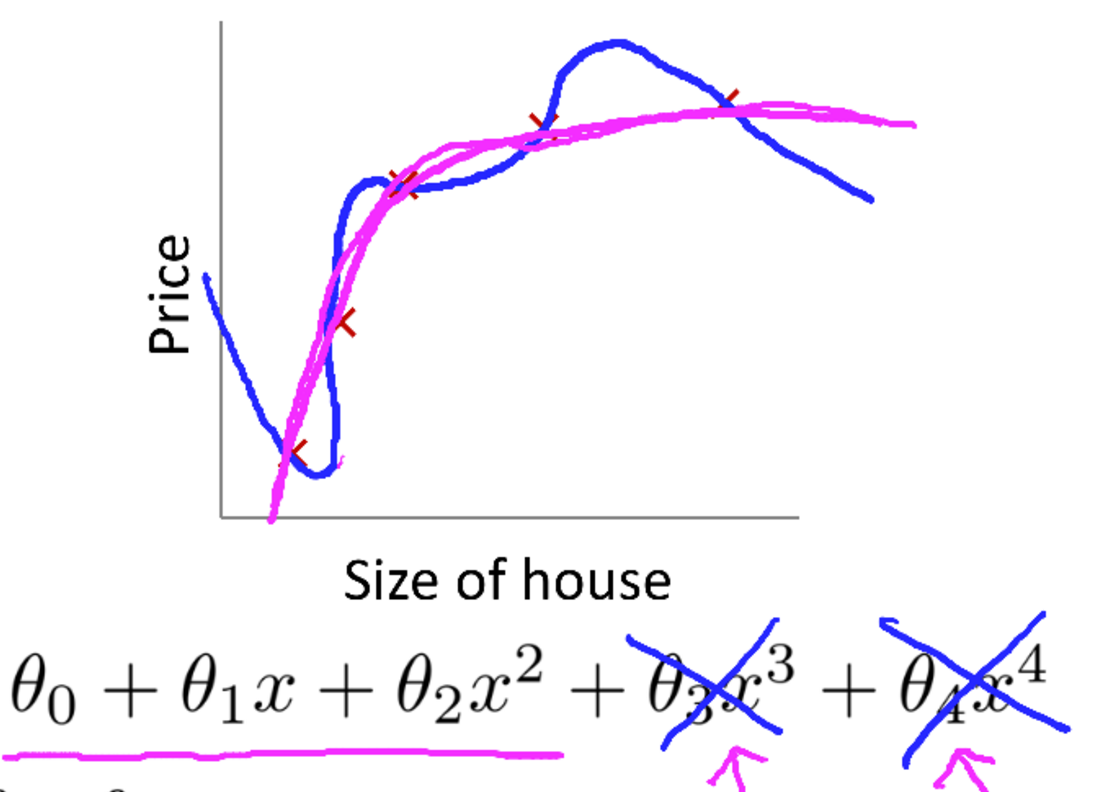## 2、线性回归模型正则化

图中蓝色线条为线性回归模型的过拟合情况，增加了${\theta }_{3}{x}^{3}$$\theta_3x^3$${\theta }_{4}{x}^{4}$$\theta_4x^4$两项后，曲线完全拟合给定样本。而红色曲线是训练的比较好的情况。在这里，我们如果想将${\theta }_{3}{x}^{3}$$\theta_3x^3$${\theta }_{4}{x}^{4}$$\theta_4x^4$从模型中剔除，可以将损失函数进行一定改造，如下所示，

$\underset{\theta }{min}\frac{1}{2m}\sum _{i=1}^{m}\left({h}_{\theta }\left({x}^{\left(i\right)}\right)-{y}^{\left(i\right)}{\right)}^{2}+1000{\theta }_{3}^{2}+1000{\theta }_{4}^{2}$

上面这个损失函数中，由于给了${\theta }_{3}$$\theta_3$${\theta }_{4}$$\theta_4$两个很大的系数，所以最终得到${\theta }_{3}$$\theta_3$${\theta }_{4}$$\theta_4$接近于0才能使损失函数值尽可能小。

正则化基本上就是这个过程，会为除${\theta }_{0}$$\theta_0$之外每个参数值增加一个类似的系数。增加了正则化后的线性回归模型损失函数如下，

$J\left(\theta \right)=\frac{1}{2m}\left[\sum _{i=1}^{m}\left({h}_{\theta }\left({x}^{\left(i\right)}\right)-{y}^{\left(i\right)}{\right)}^{2}+\lambda \sum _{j=1}^{n}{\theta }_{j}^{2}\right]$

## 3、欠拟合

假如我们给$\lambda$$\lambda$设置一个很大的参数，可能会出现过拟合的情况，因为这时候需要得到最小损失值，可能会将所有$\theta$$\theta$全部训练为0。可能最终得到的目标函数是${h}_{\theta }\left(x\right)={\theta }_{0}$$h_\theta(x)=\theta_0$，欠拟合的函数图形如下所示，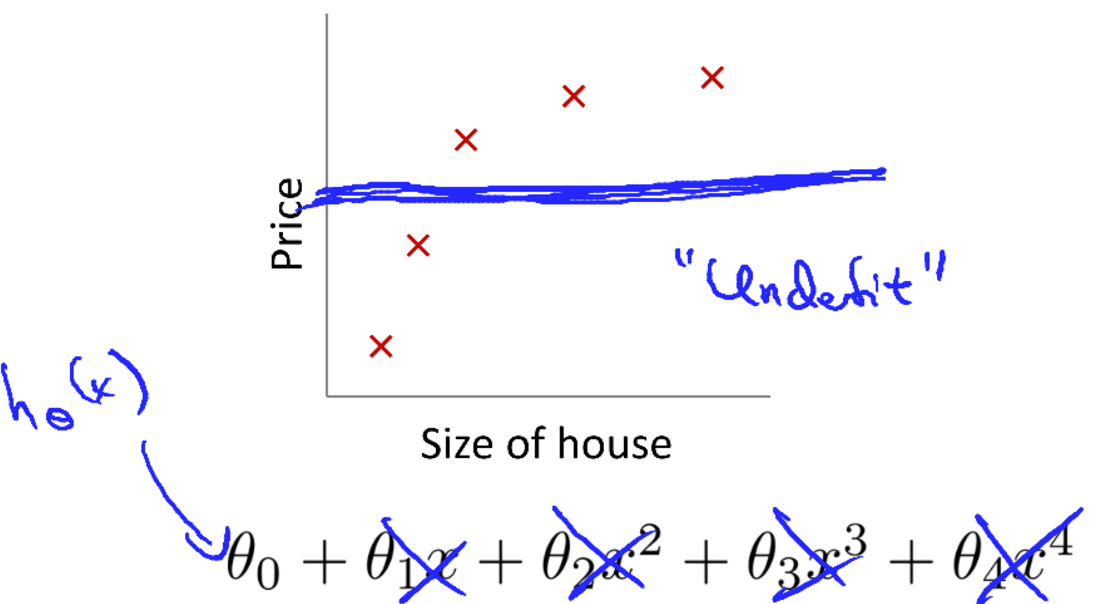## 4、线性回归模型梯度下降

对正则化之后的损失函数进行梯度下降求解参数值的过程如下所示，

这里更新${\theta }_{j}$$\theta_j$时乘以了一个系数$1-\alpha \frac{\lambda }{m}$$1-\alpha \frac\lambda m$，由于$\alpha ,\lambda ,m$$\alpha, \lambda, m$都是正数，所以该系数是一个大于零的分数，最终和之前不同的是在更新$\theta$$\theta$值时会逐渐缩小$\theta$$\theta$值。

## 5、逻辑回归模型正则化

逻辑回归模型的正则化也是在损失函数最后增加正则项，如下所示，

$J\left(\theta \right)=-\frac{1}{m}\sum _{i=1}^{m}\left[{y}^{\left(i\right)}log\left({h}_{\theta }\left({x}^{\left(i\right)}\right)\right)+\left(1-{y}^{\left(i\right)}\right)log\left(1-{h}_{\theta }\left({x}^{\left(i\right)}\right)\right)\right]+\lambda \sum _{j=1}^{n}{\theta }_{j}^{2}$

7、L1正则

8、L2正则

# 四、神经网络

## 1、神经网络结构

神经网络模型是模拟生物神经元，神经网络中每个节点可以理解成一个变量比如${x}_{i}$$x_i$，不同层之间的连接线可以理解成参数比如${\theta }_{j}$$\theta_j$。神经网络结构如下所示，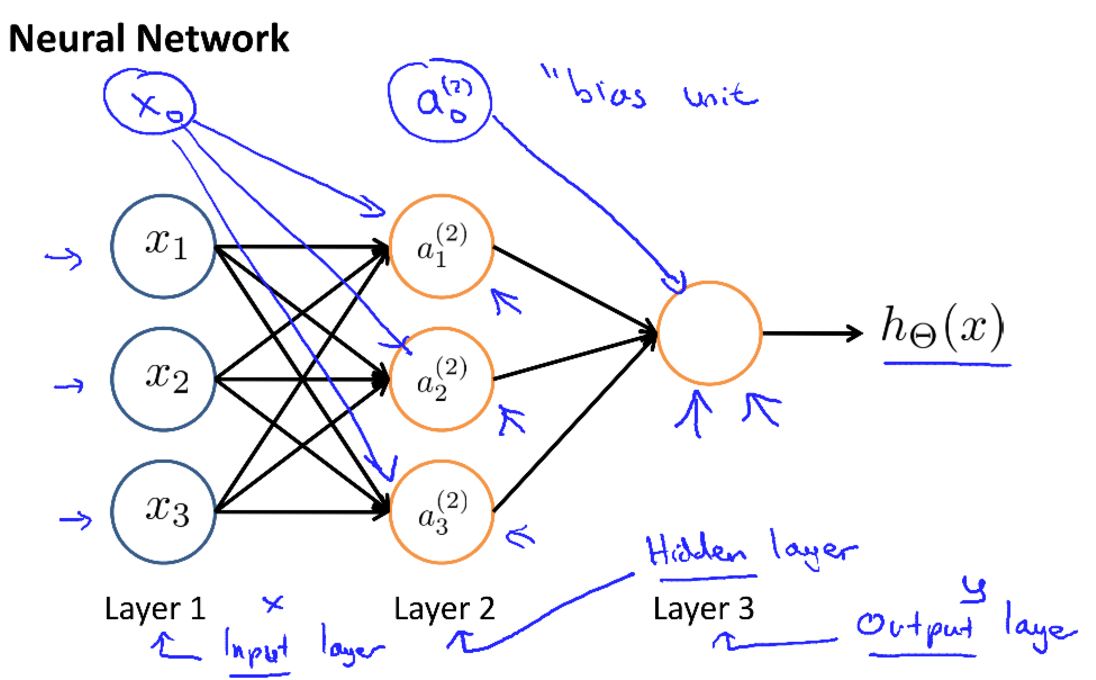上图中，第一层中的${x}_{1},{x}_{2},{x}_{3}$$x_1, x_2, x_3$即前面回归模型中见到的样本各指标值，第一层也被称为输入层，最后一层的输出就是我们前面介绍到的${h}_{\theta }\left(x\right)$$h_\theta(x)$的输出值，最后一层也被称为输出层。并且在实现神经网络模型时会为除输出层之外的每一层增加一个${x}_{0}$$x_0$或\$a_0^{(2)}这么一个偏置项。

定义几个概念：

• ${a}_{i}^{\left(j\right)}$$a_i^{(j)}$，表示第$j$$j$层的第$i$$i$个节点
• ${\mathrm{\Theta }}^{\left(j\right)}$$\Theta^{(j)}$，表示从第$j$$j$层到第$j+1$$j+1$层的参数矩阵，图中${\mathrm{\Theta }}^{\left(1\right)}$$\Theta^{(1)}$是一个$3\ast 4$$3*4$的矩阵，3表示下一层(即第2层)有3个节点，4表示本层(即第1层)有4个节点(包含${x}_{0}$$x_0$项)

生物上的神经元之间传递的电信号，一般是高低电平，而非一个连续的值。所以在我们的神经网络中一般会应用一个激活函数$g\left(x\right)$$g(x)$，以后未作特殊说明，$g\left(x\right)$$g(x)$一般取Sigmoid函数。上图中神经网络结构对应的表达式如下